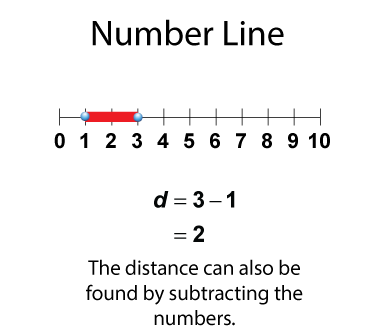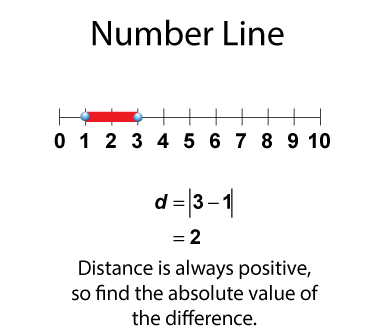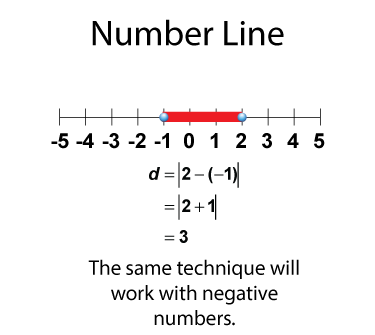# Video Tutorial: Rational Numbers: Rational Numbers on a Number Line

## Distance Measures on a Number Line

### A Brief Review of Number Lines

A number line is a horizontal line with equally spaced tic marks representing numbers.You can use a number line to represent numbers on a number line.### Distance on a Number Line

For any two numbers on a number line, you can find the distance between the two numbers.You can find the distance between the two numbers by subtracting.Distance is always positive, so find the absolute value of the difference.This technique will also work with negative numbers.This is part of a collection of video tutorials on the topic of Rational Numbers.

## Related Resources

To see resources related to this topic click on the Related Resources tab above.

## Math Video Library

To see the complete collection of these videos, click on this link.

Common Core Standards CCSS.MATH.CONTENT.6.RP.A.1, CCSS.MATH.CONTENT.7.NS.A.2.A, CCSS.MATH.CONTENT.8.NS.A.1, CCSS.MATH.CONTENT.6.NS.C.5, CCSS.MATH.CONTENT.6.NS.C.6, CCSS.Math.CONTENT.7.NS.A.1, CCSS.MATH.CONTENT.7.NS.A.1.C, CCSS.MATH.CONTENT.7.NS.A.1.D 5.10 minutes 6 - 8 Algebra     • Rational Expressions and Functions         • Rational Expressions 2017 rational numbers, rational numbers video tutorials, video tutorials, distance on a number line, video tutorial